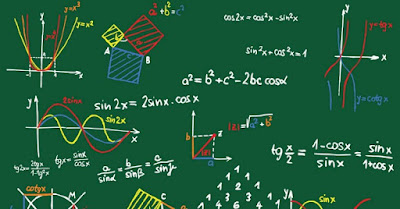## Saturday, March 30, 2019

### Interesting Facts About Number Theory | Science - Interesting Engineering

Marcia Wendorf, former high school math teacher, technical writer, author, and programmer summarizes, Among his many other accomplishments, Carl Friedrich Gauss contributed greatly to the important branch of mathematics called number theory.Photo: Pixabay
Number theory is a branch of mathematics devoted to the study of integers, the so-called counting numbers. Number theory got its start with the ancient Babylonians.

A Babylonian tablet dating to 1,800 BC contains a list of Pythagorean triples. As anyone who has ever solved for the sides of a right triangle knows, these are numbers where a2 + b2 = c2, an example being, 32 + 42 = 52

The ancient Greeks noticed a great many things about integers, for example, multiply an odd number by an even number, and the answer is always even. Then, things went dark for number theory, quite literally, as in "the dark ages."

It wasn't until French mathematician Pierre de Fermat (1607 - 1665) that number theory got a boost. Maddeningly, Fermat never published his work, and everything we know about it comes from his correspondence with other mathematicians, and in the notes he scribbled in book margins...

Contributions to Astronomy and Statistics
In 1800, the Italian astronomer Giuseppe Piazzi had discovered the dwarf planet Ceres, but it soon vanished behind the Sun before sufficient observations had been taken to calculate its orbit, and where it would reappear. Many astronomers submitted their ideas of where Ceres would reappear, but one idea differed dramatically from the rest – Gauss's.

When Ceres reappeared on December 7, 1801, it was almost exactly where Gauss had predicted it would be. To find Ceres, Gauss had invented the method of least squares. This method finds the line of best fit for a set of data, where each point of data is representative of the relationship between a known independent variable and an unknown dependent variable. Today, the method of least squares is used extensively in the financial industry.